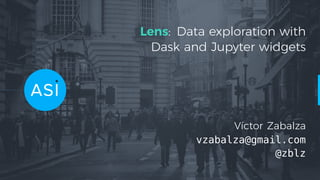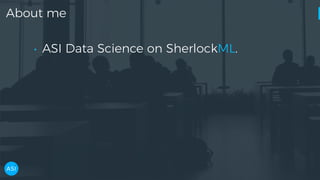Successfully reported this slideshow.

# Lens: Data exploration with Dask and Jupyter widgets×

1 of 84 Ad
1 of 84 Ad

# Lens: Data exploration with Dask and Jupyter widgets

The first step in any data-intensive project is understanding the available data. To this end, data scientists spend a significant part of their time carrying out data quality assessments and data exploration. In spite of this being a crucial step, it usually requires repeating a series of menial tasks before the data scientist gains an understanding of the dataset and can progress to the next steps in the project.

In this talk I will present Lens (https://github.com/asidatascience/lens), a Python package which automates this drudge work, enables efficient data exploration, and kickstarts data science projects. A summary is generated for each dataset, including:
- General information about the dataset, including data quality of each of the columns;
- Distribution of each of the columns through statistics and plots (histogram, CDF, KDE), optionally grouped by other categorical variables;
- 2D distribution between pairs of columns;
- Correlation coefficient matrix for all numerical columns.

Building this tool has provided a unique view into the full Python data stack, from the parallelised analysis of a dataframe within a Dask custom execution graph, to the interactive visualisation with Jupyter widgets and Plotly. During the talk, I will also introduce how Dask works, and demonstrate how to migrate data pipelines to take advantage of its scalable capabilities.

The first step in any data-intensive project is understanding the available data. To this end, data scientists spend a significant part of their time carrying out data quality assessments and data exploration. In spite of this being a crucial step, it usually requires repeating a series of menial tasks before the data scientist gains an understanding of the dataset and can progress to the next steps in the project.

In this talk I will present Lens (https://github.com/asidatascience/lens), a Python package which automates this drudge work, enables efficient data exploration, and kickstarts data science projects. A summary is generated for each dataset, including:
- General information about the dataset, including data quality of each of the columns;
- Distribution of each of the columns through statistics and plots (histogram, CDF, KDE), optionally grouped by other categorical variables;
- 2D distribution between pairs of columns;
- Correlation coefficient matrix for all numerical columns.

Building this tool has provided a unique view into the full Python data stack, from the parallelised analysis of a dataframe within a Dask custom execution graph, to the interactive visualisation with Jupyter widgets and Plotly. During the talk, I will also introduce how Dask works, and demonstrate how to migrate data pipelines to take advantage of its scalable capabilities.

### Lens: Data exploration with Dask and Jupyter widgets

1. 1. Lens: Data exploration with Dask and Jupyter widgets Víctor Zabalza vzabalza@gmail.com @zblz
2. 2. About me • ASI Data Science on SherlockML.
3. 3. About me • ASI Data Science on SherlockML. • Former astrophysicist.
4. 4. About me • ASI Data Science on SherlockML. • Former astrophysicist. • Main developer of naima, a package to model non-thermal astrophysical sources. • matplotlib developer.
5. 5. Data Exploration
6. 6. First steps in a Data Science project • Does the data ﬁt in a single computer?
7. 7. First steps in a Data Science project • Does the data ﬁt in a single computer? • Data quality assessment • Data exploration • Data cleaning
8. 8. 80% → >30 h/week
9. 9. Can we automate the drudge work?
10. 10. Developing a tool for data exploration based on Dask
11. 11. Lens Open source library for automated data exploration
12. 12. Lens by example Room occupancy dataset • ML standard dataset • Goal: predict whether room is occupied from ambient measurements.
13. 13. Lens by example Room occupancy dataset • ML standard dataset • Goal: predict whether room is occupied from ambient measurements. • What can we learn about it with Lens?
14. 14. Python interface >>> import lens
15. 15. Python interface >>> import lens >>> df = pd.read_csv('room_occupancy.csv') >>> ls = lens.summarise(df) >>> type(ls) <class 'lens.summarise.Summary'> >>> ls.to_json('room_occupancy_lens.json')
16. 16. Python interface >>> import lens >>> df = pd.read_csv('room_occupancy.csv') >>> ls = lens.summarise(df) >>> type(ls) <class 'lens.summarise.Summary'> >>> ls.to_json('room_occupancy_lens.json') room_occupancy_lens.json now contains all information needed for exploration!
17. 17. Python interface — Columns >>> ls.columns ['date', 'Temperature', 'Humidity', 'Light', 'CO2', 'HumidityRatio', 'Occupancy']
18. 18. Python interface — Categorical summary >>> ls.summary('Occupancy') {'name': 'Occupancy', 'desc': 'categorical', 'dtype': 'int64', 'nulls': 0, 'notnulls': 8143, 'unique': 2} >>> ls.details('Occupancy') {'desc': 'categorical', 'frequencies': {0: 6414, 1: 1729}, 'name': 'Occupancy'}
19. 19. Python interface — Numeric summary >>> ls.details('Temperature') {'name': 'Temperature', 'desc': 'numeric', 'iqr': 1.69, 'min': 19.0, 'max': 23.18, 'mean': 20.619, 'median': 20.39, 'std': 1.0169, 'sum': 167901.1980}
20. 20. The lens.Summary is a good building block, but clunky for exploration. Can we do better?
21. 21. Jupyter widgets
22. 22. Jupyter widgets: Column distribution
23. 23. Jupyter widgets: Correlation matrix
24. 24. Jupyter widgets: Pair density
25. 25. Jupyter widgets: Pair density
26. 26. Building Lens
27. 27. Our solution: Analysis • A Python function computes dataset metrics: • Column-wise statistics • Pairwise densities • ...
28. 28. Our solution: Analysis • A Python function computes dataset metrics: • Column-wise statistics • Pairwise densities • ... • Computation cost is paid up front. • The result is serialized to JSON.
29. 29. Our Solution: Interactive exploration • Using only the report, the user can explore the dataset through either: • Jupyter widgets • Web UI
30. 30. The lens Python library
31. 31. Why Python? • Data Scientists
32. 32. Why Python? • Data Scientists • Portability • Reusability
33. 33. Why Python? • Data Scientists • Portability • Reusability • Scalability
34. 34. Why Python? • Data Scientists • Portability • Reusability • Scalability Can Python scale?
35. 35. Out-of-core options in Python Difficult Flexible Easy Restrictive
36. 36. Out-of-core options in Python Difficult Flexible Easy Restrictive Threads, Processes, MPI, ZeroMQ Concurrent.futures, joblib
37. 37. Out-of-core options in Python Difficult Flexible Easy Restrictive Threads, Processes, MPI, ZeroMQ Concurrent.futures, joblib Luigi PySpark Hadoop, SQL
38. 38. Out-of-core options in Python Difficult Flexible Easy Restrictive Threads, Processes, MPI, ZeroMQ Concurrent.futures, joblib Luigi PySpark Hadoop, SQL
39. 39. Out-of-core options in Python Difficult Flexible Easy Restrictive Threads, Processes, MPI, ZeroMQ Concurrent.futures, joblib Luigi PySpark Hadoop, SQL Dask
41. 41. Dask interface • Dask objects are lazily computed.
42. 42. Dask interface • Dask objects are lazily computed. • The user operates on them as Python structures.
43. 43. Dask interface • Dask objects are lazily computed. • The user operates on them as Python structures. • Dask builds a DAG of the computation.
44. 44. Dask interface • Dask objects are lazily computed. • The user operates on them as Python structures. • Dask builds a DAG of the computation. • DAG is executed when a result is requested.
45. 45. dask.delayed — Build your own DAG
46. 46. dask.delayed — Build your own DAG files = ['myfile.a.data', 'myfile.b.data', 'myfile.c.data'] loaded = [load(i) for i in files] cleaned = [clean(i) for i in loaded] analyzed = analyze(cleaned) store(analyzed)
47. 47. dask.delayed — Build your own DAG @delayed def load(filename): ... @delayed def clean(data): ... @delayed def analyze(sequence_of_data): ... @delayed def store(result): with open(..., 'w') as f: f.write(result)
48. 48. dask.delayed — Build your own DAG files = ['myfile.a.data', 'myfile.b.data', 'myfile.c.data'] loaded = [load(i) for i in files] cleaned = [clean(i) for i in loaded] analyzed = analyze(cleaned) stored = store(analyzed)
49. 49. dask.delayed — Build your own DAG files = ['myfile.a.data', 'myfile.b.data', 'myfile.c.data'] loaded = [load(i) for i in files] cleaned = [clean(i) for i in loaded] analyzed = analyze(cleaned) stored = store(analyzed) clean-2 analyze cleanload-2 analyze store clean-3 clean-1 load storecleanload-1 cleanload-3load load
50. 50. dask.delayed — Build your own DAG files = ['myfile.a.data', 'myfile.b.data', 'myfile.c.data'] loaded = [load(i) for i in files] cleaned = [clean(i) for i in loaded] analyzed = analyze(cleaned) stored = store(analyzed) clean-2 analyze cleanload-2 analyze store clean-3 clean-1 load storecleanload-1 cleanload-3load load stored.compute()
51. 51. Dask high-level collections These implement a good fraction of the APIs of their Python counterparts: • dask.array • dask.dataframe • dask.bag
52. 52. Dask tools for Machine Learning • dask-ml • dask-searchcv • dask-XGBoost • dask-tensorflow • ...
53. 53. Dask schedulers — How is the graph executed? • Synchronous — good for testing
54. 54. Dask schedulers — How is the graph executed? • Synchronous — good for testing • Threaded — I/O and GIL-releasing code
55. 55. Dask schedulers — How is the graph executed? • Synchronous — good for testing • Threaded — I/O and GIL-releasing code • Multiprocessing — bypass GIL
56. 56. Dask schedulers — How is the graph executed? • Synchronous — good for testing • Threaded — I/O and GIL-releasing code • Multiprocessing — bypass GIL • Distributed — run in multiple nodes
57. 57. Dask DAG execution
58. 58. Dask DAG execution In memory Released from memory
59. 59. Dask DAG execution In memory Released from memory
60. 60. How do we use Dask in Lens?
61. 61. Lens pipeline DataFrame colA colB
62. 62. Lens pipeline DataFrame colA colB PropA PropB
63. 63. Lens pipeline DataFrame colA colB PropA PropB SummA SummB
64. 64. Lens pipeline DataFrame colA colB PropA PropB SummA SummB OutA OutB
65. 65. Lens pipeline DataFrame colA colB PropA PropB SummA SummB OutA OutB Corr
66. 66. Lens pipeline DataFrame colA colB PropA PropB SummA SummB OutA OutB Corr PairDensity
67. 67. Lens pipeline DataFrame colA colB PropA PropB SummA SummB OutA OutB Corr PairDensity Report
68. 68. • Graph for two-column dataset generated by lens.
69. 69. • Graph for two-column dataset generated by lens. • The same code can be used for much wider datasets.
70. 70. Integration with SherlockML
71. 71. SherlockML integration • Every dataset entering the platform is analysed by Lens.
72. 72. SherlockML integration • Every dataset entering the platform is analysed by Lens. • We can use the same Python library!
73. 73. SherlockML integration • Every dataset entering the platform is analysed by Lens. • We can use the same Python library! • The web frontend is used to interact with datasets.
74. 74. SherlockML: Column information
75. 75. SherlockML: Column distribution
76. 76. SherlockML: Correlation matrix
77. 77. SherlockML: Pair density
78. 78. Data Exploration with Lens
79. 79. Data Exploration with Lens • Scalable compute with dask.
80. 80. Data Exploration with Lens • Scalable compute with dask. • Snappy interactive exploration.
81. 81. Data Exploration with Lens • Scalable compute with dask. • Snappy interactive exploration. • Lens is open source: • GitHub: ASIDataScience/lens • Docs: https://lens.readthedocs.io • PyPI: pip install lens